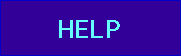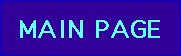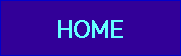Exploration #1Question: What is the Mandelbrot set?

Answer: The Mandelbrot set is a picture in the complex "c-plane" of the fate of the orbit of 0 under iteration of the function x2 + c. A c-value is in the Mandelbrot set if the orbit of 0 under iteration of x2 + c for the particular value of c does not tend to infinity. If the orbit of 0 tends to infinity, then that c-value is not in the Mandelbrot set.Question: Hey man, slow down! Wanna give me that in English? (please...)

Answer: OK, here we go again. You give me a complex number, call it c. Then I will tell you whether c is in the Mandelbrot set. OK? Here's how I do it. I start with the function x2 + c. Then I take 0 and put it into the function. That is, I compute 02 + c which gives c. Then I do this again, using the output from the previous computation as the input for the next. That is, I now use c and compute c2 + c. Then I do it again: I get (c2 + c)2 + c. And so forth. I generate a list of complex numbers in this way. If these complex numbers (called the orbit of 0) get larger and larger (or further and further away from the origin), then your choice of c is NOT in the Mandelbrot set. But if this is not the case (the orbit stays "bounded"), then c IS in the Mandelbrot set. Are you happy now?

In each of the following five examples, observe the following information:

• The location of c in or around the Mandelbrot set.
• The list of the first few points on the orbit of 0.
• The location of this orbit in the complex plane.

Example 1: c = 0 .

Question 1A: Is c = 3 in the Mandelbrot set? Answer

Question 1B: Is c = 1 in the Mandelbrot set? Answer

Question 1C: Is c = 1 + i in the Mandelbrot set? Answer

Thus we see that the following c-values are in the Mandelbrot set:

• c = 0
• c = i
• c = -2
• c = -1
whereas the following c-values are not in the Mandelbrot set:
• c = 2i
• c = 3
• c = 1
• c = 1 + i

We may therefore begin to "paint" the picture of the Mandelbrot set by coloring complex numbers in the c-plane according to the following rule:

• Color a c-value black if c lies in the Mandelbrot set (that is, the corresponding orbit of c does not escape to infinity.

• Color a c-value a different color if the orbit escapes to infinity. In the Explorer, we always use the following algorithm to color points: red points escape fastest. That is, it takes very few iterations for the orbit of 0 under iteration of x2 + c to become far from the origin. Red points are followed in order by orange, yellow, green, blue, indigo, and violet. That is we use the colors drawn from the light spectrum for points that are not in the Mandelbrot set. The important thing is: red points escape fastest, while violet points take the most iterations to go far away from the origin.

Thus we have the following picture so far:Further Exploration:

The Java applet below allows you to click on a point in or around the Mandelbrot set and then view the fate of the orbit of 0. By fate of the orbit we mean the eventual behavior of the orbit of 0: We do not plot all of the points on the orbit of 0. We merely display the points number 25, 26, 27, etc. on the orbit of 0.

• In many cases the orbit of 0 tends to a cycle of period n. In this case we display that cycle as well as its period.
• Sometimes the orbit of 0 does not tend to a cycle or tends to a cycle with an extremely large period that we cannot compute in a reasonable amount of time. In this case we report the fate of the orbit as "cannot determine period."
• If the orbit tends to infinity, we report "goes to infinity" and do not plot the orbit.